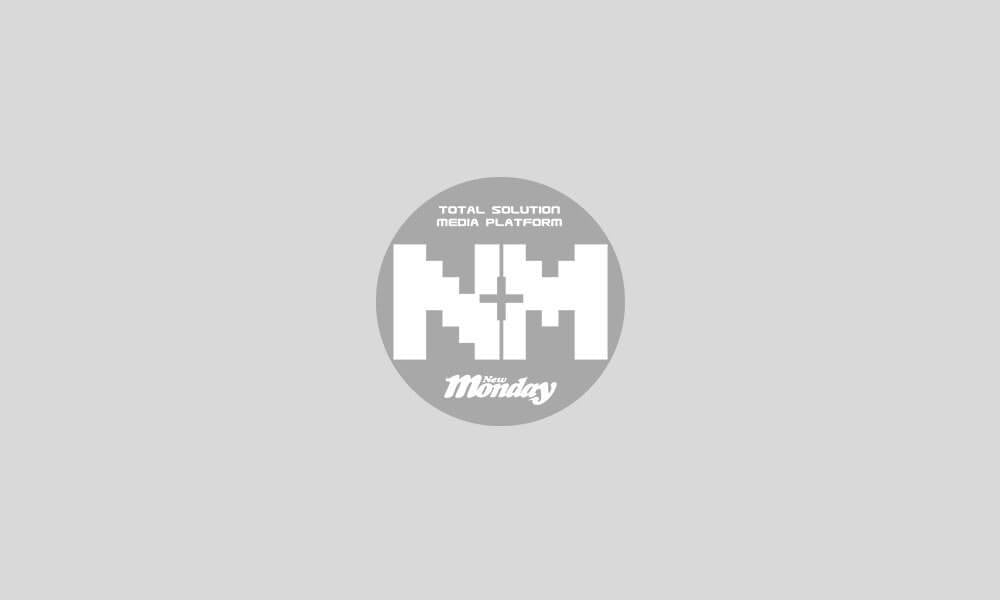# AFAIK？BBT？GL？到底係點解？ 教你40個常見WhatsApp潮語

## 常用詞彙

AFAIK = As far as I know = 就我所知
AFK = Away from keyboard = 暫時離開
AKA = As known as = 也就是
BBT = Be back tomorrow = 明天回來
BRB = Be right back = 馬上回來
BOT = Back on topic = 回到主題FYI = For your information = 給你的資訊
GL = Good luck = 祝你好運
grats = Congratulations = 恭喜

IDK = I don’t know = 我不知道
IMO = In my opinion 在我看來
LMK = Let me know = 話我知NP = No problem = 沒問題
ORLY = Oh really = 真的嗎
PPL = People = 大家
RTG = Ready to go = 準備出發
TBH = To be honest = 老實說
OMW = On my way = 在路上

TGIF = Thank God is Friday = 感謝主終於到星期五了
TTYL = Talk to you later = 遲啲傾
TKS = Thanks = 謝謝
LOL = Laugh out loud = 笑到好大聲
ROFL = Roll on floor laughing = 笑到碌地
OMG = Oh my God = 我的天## 簡化字句

ASAP = As soon as possible = 愈快愈好
ATM = At the moment = 現在
B-DAY = Birthday = 生日

EA = Each = 每個
HAV = Have = 有
NPNT = No picture / photo no truth 冇圖冇真相
PM = Private message = 私人留言
RIP = Rest in peace = 安息
TMR = Tomorrow = 明日ahaha = 啊哈哈
er = 呃……
hm = 嗯……
oops = 弊喇！
oh = 喔
yeah/ yup = 同意